## 6.3.11 PostFilter

### 6.3.11.1 Outline of the node

This node performs postprocessing to improve the accuracy of speech recognition with the sound source separation node GHDSS for a separated complex spectrum. At the same time, it generates noise power spectra to generate Missing Feature Masks.

### 6.3.11.2 Necessary files

No files are required.

### 6.3.11.3 Usage

When to use

This node is used to form the spectrum that are separated by the GHDSS node and generate the noise spectra required to generate Missing Feature Masks.

Typical connection

Figure 670 shows an example of a connection for the PostFilter node. The output of the GHDSS node is connected to the INPUT_SPEC input and the output of the BGNEstimator node is connected to the INIT_NOISE_POWER input. Figure 670 shows examples for typical output connections:

1. Speech feature extraction from separated sound (OUTPUT_SPEC) (MSLSExtraction node)

2. Generation of Missing Feature Masks from separated sound and power (EST_NOISE_POWER) of noise contained in it at the time of speech recognition (MFMGeneration node)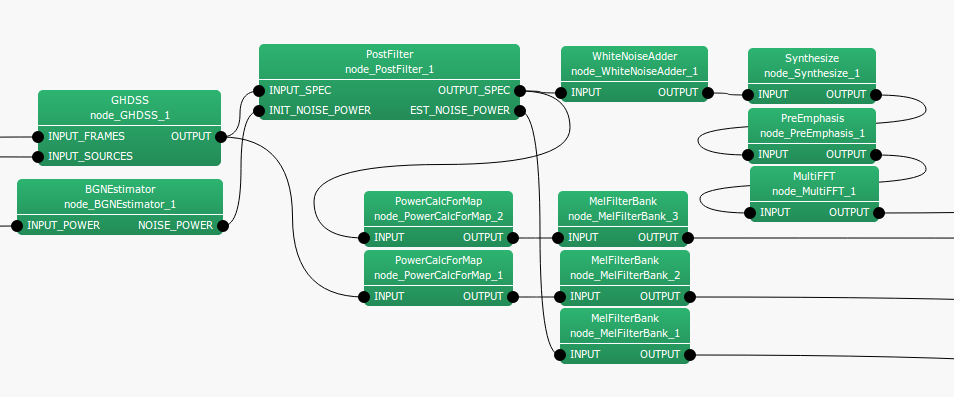### 6.3.11.4 Input-output and property of the node

Input

INPUT_SPEC

: Map<int, ObjectRef> type. The same type as the output from the GHDSS node. A pair of a sound source ID and a complex spectrum of the separated sound as Vector<complex<float> > type data.

INPUT_NOISE_POWER

: Matrix<float> type. The power spectrum of the stationary noise estimated by the BGNEstimator node.

Output

OUTPUT_SPEC

: Map<int, ObjectRef> type. The Object is the complex spectrum from the input INPUT_SPEC, with noise removed.

EST_NOISE_POWER

: Map<int, ObjectRef> type. Power of the estimated noise to be contained is paired with IDs as Vector<float> type data for each separated sound of OUTPUT_SPEC.

Parameter

Table 6.63: Parameter list of PostFilter (first half)
 Parameter name Type Default value Description MCRA_SETTING false When the user set parameters for the MCRA estimation, which is a noise removal method, select true. MCRA_SETTING The following are valid when MCRA_SETTING is set to true STATIONARY_NOISE_FACTOR 1.2 Coefficient at the time of stationary noise estimation. SPEC_SMOOTH_FACTOR 0.5 Smoothing coefficient of an input power spectrum. AMP_LEAK_FACTOR 1.5 Leakage coefficient. STATIONARY_NOISE_MIXTURE_FACTOR 0.98 Mixing ratio of stationary noise. LEAK_FLOOR 0.1 Minimum value of leakage noise. BLOCK_LENGTH 80 Detection time width. VOICEP_THRESHOLD 3 Threshold value of speech presence judgment. EST_LEAK_SETTING false When the user sets parameters related to the leakage rate estimation, select true. EST_LEAK_SETTING The followings are valid when EST_LEAK_SETTING is set to true. LEAK_FACTOR 0.25 Leakage rate. OVER_CANCEL_FACTOR 1 Leakage rate weighting factor. EST_REV_SETTING false When the user sets parameters related to the component estimation, select true. EST_REV_SETTING The followings are valid when EST_REV_SETTING is set to true. REVERB_DECAY_FACTOR 0.5 Damping coefficient of reverberant power. DIRECT_DECAY_FACTOR 0.2 Damping coefficient of a separated spectrum. EST_SN_SETTING false When the user sets parameters related to the SN ratio estimation, select true. EST_SN_SETTING The followings are valid when EST_SN_SETTING is set to true. PRIOR_SNR_FACTOR 0.8 Ratio of priori and posteriori SNRs. VOICEP_PROB_FACTOR 0.9 Amplitude coefficient of the probability of speech presence. MIN_VOICEP_PROB 0.05 Probability of the minimum speech presence. MAX_PRIOR_SNR 100 Maximum value of preliminary SNR. MAX_OPT_GAIN 20 Maximum value of the optimal gain intermediate variable v. MIN_OPT_GAIN 6 Minimum value of the optimal gain intermediate variable v.
Table 6.64: Parameter list of PostFilter (latter half)
 Parameter name Type Default value Description EST_VOICEP_SETTING false When the user sets parameters related to the speech probability estimation, select true. EST_VOICEP_SETTING The following are valid when EST_VOICEP_SETTING is set to true. PRIOR_SNR_SMOOTH_FACTOR 0.7 Time smoothing coefficient. MIN_FRAME_SMOOTH_SNR 0.1 Minimum value of the frequency smoothing SNR (frame). MAX_FRAME_SMOOTH_SNR 0.316 Maximum value of the frequency smoothing SNR (frame). MIN_GLOBAL_SMOOTH_SNR 0.1 Minimum value of the frequency smoothing SNR (global). MAX_GLOBAL_SMOOTH_SNR 0.316 Maximum value of the frequency smoothing SNR (global). MIN_LOCAL_SMOOTH_SNR 0.1 Minimum value of the frequency smoothing SNR (local). MAX_LOCAL_SMOOTH_SNR 0.316 Maximum value of the frequency smoothing SNR (local). UPPER_SMOOTH_FREQ_INDEX 99 Frequency smoothing upper limit bin index. LOWER_SMOOTH_FREQ_INDEX 8 The frequency smoothing lower limit bin index. GLOBAL_SMOOTH_BANDWIDTH 29 Frequency smoothing band width (global). LOCAL_SMOOTH_BANDWIDTH 5 The frequency smoothing band width (local). FRAME_SMOOTH_SNR_THRESH 1.5 Threshold value of frequency smoothing SNR. MIN_SMOOTH_PEAK_SNR 1.0 Minimum value of the frequency smoothing SNR peak. MAX_SMOOTH_PEAK_SNR 10.0 Maximum value of the frequency smoothing SNR peak. FRAME_VOICEP_PROB_FACTOR 0.7 Speech probability smoothing coefficient (frame). GLOBAL_VOICEP_PROB_FACTOR 0.9 Speech probability smoothing coefficient (global). LOCAL_VOICEP_PROB_FACTOR 0.9 Speech probability smoothing coefficient (local). MIN_VOICE_PAUSE_PROB 0.02 Minimum value of speech quiescent probability. MAX_VOICE_PAUSE_PROB 0.98 Maximum value of speech quiescent probability.

### 6.3.11.5 Details of the node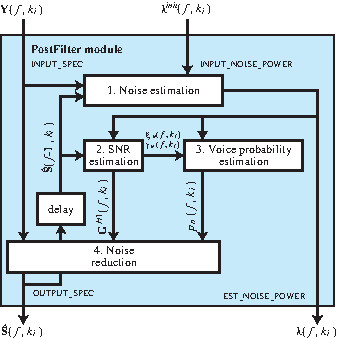Figure 6.75: Flowchart of PostFilter

The subscripts used in the equations are based on the definitions in Table 6.1. Moreover, the time frame index $f$ is abbreviated in the following equations unless especially needed. Figure 6.75 shows a flowchart of the PostFilter node. A separated sound spectrum from the GHDSS node and a stationary noise power spectrum of the BGNEstimator node are obtained as inputs. Outputs are the separated sound spectrum for which the speech is emphasized, and a power spectrum of noise mixed with the separated sound. The processing flow is as follows.

1. Noise estimation

2. SNR estimation

3. Speech presence probability estimation

4. Noise removal

1) Noise estimation: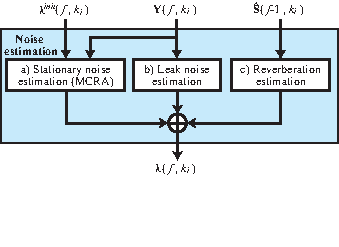Figure 6.76: Procedure of noise estimation

Figure 6.76 shows the processing flow of noise estimation . The three kinds of noise that the PostFilter node processes are:
a) The stationary noise for which contact points of microphones are a factor,
b) The sound of other sound sources that cannot be completely removed (leakage noise),
c) Reverberations from the previous frame.

The noise contained in the final separated sound $\boldsymbol {\lambda }(f, k_ i)$ is obtained by the following equation.

 $\displaystyle \boldsymbol {\lambda }(f,k_ i)$ $\displaystyle =$ $\displaystyle \boldsymbol {\lambda }^{sta}(f,k_ i) + \boldsymbol {\lambda }^{leak}(f,k_ i) + \boldsymbol {\lambda }^{rev}(f-1,k_ i)$ (91)

Here, $\boldsymbol {\lambda }^{sta}(f,k_ i), \boldsymbol {\lambda }^{leak}(f,k_ i)$ and $\boldsymbol {\lambda }^{rev}(f-1,k_ i)$ indicate stationary noise, leakage noise and reverberation from the previous frame, respectively.

#### 6.3.11.5.1 1-a) Stationary noise estimation by MCRA method

The parameters used in 1-a) are based on Table 6.65.

Table 6.65: Definition of variable
 Parameter Description, Corresponding parameter $\boldsymbol {Y}(k_ i) = \left[Y_1(k_ i),\dots , Y_ N(k_ i) \right]^ T$ Complex spectrum of separated sound corresponding to the frequency bin $k_ i$ $\boldsymbol {\lambda }^{init}(k_ i) = \left[\lambda ^{init}_{1}(k_ i),\dots , \lambda ^{init}_ N(k_ i)\right]^ T$ Initial value power spectrum used for the stationary noise estimation $\boldsymbol {\lambda }^{sta}(k_ i) = \left[\lambda ^{sta}_{1}(k_ i),\dots , \lambda ^{sta}_ N(k_ i) \right]^ T$ Estimated stationary noise power spectrum. $\alpha _ s$ Smoothing coefficient of the input power spectrum. Parameter SPEC_SMOOTH_FACTOR. The default value is 0.5 $\boldsymbol {S}^{tmp}(k_ i)= \left[S^{tmp}_1(k_ i),\dots , S^{tmp}_ N(k_ i) \right]$ Temporary parameter for minimum power calculation. $\boldsymbol {S}^{min}(k_ i)= \left[S^{min}_1(k_ i),\dots , S^{min}_ N(k_ i) \right]$ The parameter that maintains the minimum power. $L$ Maintained frame numbers of $\boldsymbol {S}_{tmp}$. Parameter BLOCK_LENGTH. The default value is 80 $\delta$ Threshold value of speech presence judgment. Parameter VOICEP_THRESHOLD. The default value is 3.0 $\alpha _ d$ Mixing ratio of estimated stationary noise. Parameter STATIONARY_NOISE_MIXTURE_FACTOR. The default value is 0.98 $\boldsymbol {Y}^{leak}(k_ i)$ Power spectrum of leakage noise estimated, to be contained in separated sound $q$ Coefficient for when leakage noise is removed from the input separated sound power. Parameter AMP_LEAK_FACTOR. The default value is 1.5. $S_{floor}$ Minimum value of leakage noise. Parameter LEAK_FLOOR. The default value is 0.1. $r$ Coefficient at the time of stationary noise estimation. Parameter STATIONARY_NOISE_FACTOR. The default value is 1.2

First, calculate the power spectrum for which the input spectrum is smoothed with the power from one frame before. $\boldsymbol {S}(f,k_ i) = \left[S_1(f,k_ i),\dots , S_ N(f,k_ i)\right]$.

 $\displaystyle S_ n(f,k_ i)$ $\displaystyle =$ $\displaystyle \alpha _ s S_ n(f-1,k_ i)+ (1 - \alpha _ s)|Y_ n(k_ i)|^2 \label{eq:MCRA-smooth}$ (92)

Next, update $\boldsymbol {S}^{tmp}$, $\boldsymbol {S}^{min}$.

 $\displaystyle S^{min}_ n(f,k_ i)$ $\displaystyle =$ $\displaystyle \left\{ \begin{array}{cr} \min \{ S^{min}_ n(f-1,k_ i),S_ n(f,k_ i) & \mathrm{if}\ \ f \neq nL\\ \min \{ S^{tmp}_ n(f-1,k_ i),S_ n(f,k_ i) & \mathrm{if}\ \ f = nL \end{array}\right.,$ (93) $\displaystyle S^{min}_ n(f,k_ i)$ $\displaystyle =$ $\displaystyle \left\{ \begin{array}{cr} \min \{ S^{tmp}_ n(f-1,k_ i),S_ n(f,k_ i) & \mathrm{if}\ \ f \neq nL\\ S_ n(f,k_ i) & \mathrm{if}\ \ f = nL \end{array}\right.,$ (94)

Here, $n$ indicates an arbitrary integer. $\boldsymbol {S}^{min}$ maintains the minimum power after the noise estimation begins $\boldsymbol {S}^{tmp}$ maintains an extremely small power of a recent frame. $\boldsymbol {S}^{tmp}$ is updated every $L$ frames. Next, judge if the frame contains speech based on the power ratio of the minimum power and the input separated sound.

 $\displaystyle S_ n^{r}(k_ i)$ $\displaystyle =$ $\displaystyle \frac{S_ n(k_ i)}{S^{min}(k_ i)},$ (95) $\displaystyle I_ n(k_ i)$ $\displaystyle =$ $\displaystyle \left\{ \begin{array}{cr} 1 & \mathrm{if}\ \ S_ n^ r(k_ i) > \delta \\ 0 & \mathrm{if}\ \ S_ n^ r(k_ i) \leq \delta \end{array} \right.$ (96)

When speech is included, $I_ n(k_ i)$ is 1 and when it is not included, it is 0. Based on this result, we determine the mixing ratio $\alpha _{d,n}^ C(k_ i)$ of the frame’s estimated stationary noise.

 $\displaystyle \alpha _{d,n}^ C(k_ i)$ $\displaystyle =$ $\displaystyle (\alpha _ d - 1)I_ n(k_ i)+ 1.$ (97)

Next, subtract leakage noise contained in the power spectrum of the separated sound.

 $\displaystyle S^{leak}_ n(k_ i)$ $\displaystyle =$ $\displaystyle \sum _{p=1}^{N}|Y_ p(k_ i)|^2 - |Y_ n(k_ i)|^2,\label{eq:MCRA-leak}$ (98) $\displaystyle S_ n^0(k_ i)$ $\displaystyle =$ $\displaystyle |Y_ n(k_ i)|^2 - q S^{leak}_ n(k_ i),$ (99)

Here, when $S_ n^0(k_ i) < S_{floor}$, the valued is changed to below.

 $\displaystyle S_ n^0(k_ i)$ $\displaystyle =$ $\displaystyle S_{floor}$ (100)

Obtain stationary noise of the current frame by mixing the power spectrum with leakage noise removed $S_ n^0(f,k_ i)$ and the estimated stationary noise of the former frame $\boldsymbol {\lambda }^{sta}(f-1,k_ i)$ or ${bf \lambda }^{init}(f,k_ i)$, which is the output from BGNEstimator .

 $\displaystyle \lambda ^{sta}_ n(f,k_ i)$ $\displaystyle =$ $\displaystyle \left\{ \begin{array}{cr} \alpha _{d,n}^ C(k_ i) \lambda ^{sta}_ n(f-1,k_ i)+ (1-\alpha _{d,n}^ C(k_ i) r S_ n^0(f,k_ i) & no change in source position\\ \alpha _{d,n}^ C(k_ i) \lambda ^{init}_ n(f,k_ i) + (1-\alpha _{d,n}^ C(k_ i) r S_ n^0(f,k_ i) & \mathrm{if }{Change in source position} \end{array} \right.$ (101)

#### 6.3.11.5.2 1-b)Leakage noise estimation

The variables used in 1-b) are based on Table 6.66.

Table 6.66: Definition of variable
 Variable Description, Corresponding parameter $\boldsymbol {\lambda }^{leak}(k_ i)$ Power spectrum of leakage noise. Vector comprising elements of each separated sound. $\alpha ^{leak}$ Leakage rate for the total of separated sound power. LEAK_FACTOR $\times$ OVER_CANCEL_FACTOR $S_ n(f,k_ i)$ Smoothing power spectrum obtained by Equation (92)

Some parameters are calculated as follows.

 $\displaystyle \beta$ $\displaystyle =$ $\displaystyle -\frac{\alpha ^{leak}}{1-(\alpha ^{leak})^2+\alpha ^{leak}(1-\alpha ^{leak})(N-2)}$ (102) $\displaystyle \alpha$ $\displaystyle =$ $\displaystyle 1 - (N-1)\alpha ^{leak}\beta$ (103)

With this parameter, mix the smoothed spectrum ${\boldsymbol {$S$}}(k_ i)$, the power spectrum for which the power of the own separated sound is removed from the power of other separated sound $S^{leak}_ n(k_ i)$ obtained by Equation (98).

 $\displaystyle Z_ n(k_ i)$ $\displaystyle =$ $\displaystyle \alpha S_ n(k_ i)+ \beta S^{leak}_ n(k_ i),$ (104)

Here, when $Z_ n(k_ i) < 1$, assume $Z_ n(k_ i) = 1$. The power spectrum of final leakage noise $\boldsymbol {\lambda }^{leak}(k_ i)$ is obtained as follows.

 $\displaystyle \lambda ^{leak}_ n$ $\displaystyle =$ $\displaystyle \alpha ^{leak} \left(\sum _{n' \neq n}Z_{n'}(k_ i) \right)$ (105)

#### 6.3.11.5.3 1-c) Reverberant estimation

The variables used in 1-c) are based on Table 6.67.

Table 6.67: Definition of variable
 Variable Description, Corresponding parameter $\boldsymbol {\lambda }^{rev}(f,k_ i)$ Power spectrum of reverberant in the time frame $f$ $\hat{\boldsymbol {S}}(f-1,k_ i)$
 $\displaystyle \lambda ^{leak}_ n$ $\displaystyle =$ $\displaystyle \alpha ^{leak} \left(\sum _{n' \neq n}Z_{n'}(k_ i) \right)$ (106)
 $\displaystyle \lambda _ n^{rev}(f,k_ i)$ $\displaystyle =$ $\displaystyle \gamma \left(\lambda _ n^{rev}(f-1,k_ i)+ \Delta |{\hat S}_ n(f-1,k_ i)|^2 \right)$ (107)

2)SNR estimation: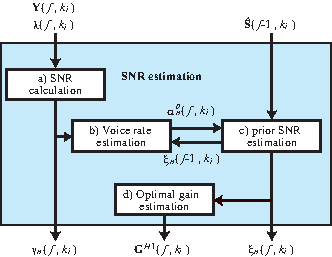Figure 6.77: Procedure of SNR estimation

Figure 6.77 shows the flow of the SNR estimation. The SNR estimation consists of the followings
a) Calculation of SNR
b) Preliminary SNR estimation before noise mixture
c) Estimation of a speech content rate
d) Estimation of an optimal gain

Table 6.68: Definition of major variable
 Variable Description, corresponding parameter $\boldsymbol {Y}(k_ i)$ Complex spectra of the separated sound, which is an input of the PostFilter node $\hat{\boldsymbol {S}}(k_ i)$ Complex spectra of the formed separated sound, which is an output of the PostFilter node $\boldsymbol {\lambda }(k_ i)$ Power spectrum of noise estimated above $\gamma _ n(k_ i)$ SNR of the separated sound $n$ $\alpha _ n^ p(k_ i)$ Speech content rate $\xi _ n(k_ i)$ Preliminary SNR $\boldsymbol {G}^{H1}(k_ i)$ Optimal gain to improve SNR of the separated sound

The vector elements in Table 6.68 indicate value of each separated sound.

#### 6.3.11.5.4 2-a) Calculation of SNR

The variables used in 2-a) are based on Table 6.68. Here, SNR $\gamma _ n(k_ i)$ is calculated based on the complex spectra $\boldsymbol {Y}(k_ i)$ of the input and the power spectrum of the noise estimated above.

 $\displaystyle \gamma _ n(k_ i)$ $\displaystyle =$ $\displaystyle \frac{|Y_ n(k_ i)|^2}{\lambda _ n(k_ i)}$ (108) $\displaystyle \gamma _ n^ C(k_ i)$ $\displaystyle =$ $\displaystyle \left\{ \begin{array}{cr} \gamma _ n(k_ i) & \mathrm{if}\ \ \gamma _ n(k_ i)> 0\\ 0 & \mathrm{otherwise} \end{array} \right.$ (109)

Here, when $\gamma _ n(k_ i) < 0$ is satisfied, $\gamma _ n(k_ i) = 0$.

#### 6.3.11.5.5 2-b)Estimation of speech content rate

The variables used in 2-b) are based on Table 6.69.

Table 6.69: Definition of variable
 Variable Description, corresponding parameter $\alpha ^ p_{mag}$ Preliminary SNR coefficient. Parameter VOICEP_PROB_FACTOR. The default value is 0.9. $\alpha ^ p_{min}$ Minimum speech content rate. Parameter MIN_VOICEP_PROB. The default value is 0.05.

The speech content rate $\alpha _ n^ p(f,k_ i)$ is calculated as follows, with the preliminary SNR $\xi _ n(f-1,k_ i)$ of the former frame.

 $\displaystyle \alpha _ n^ p(f,k_ i)$ $\displaystyle =$ $\displaystyle \alpha ^ p_{mag} \left(\frac{\xi _ n(f-1,k_ i)}{\xi _ n(f-1,k_ i)+1}\right)^2 + \alpha ^ p_{min}$ (110)

#### 6.3.11.5.6 2-c) Preliminary SNR estimation before noise mixture

The variables used in 2-c) are based on Table 6.70.

Table 6.70: Definition of variable
 Variable Description, corresponding parameter $a$ Internal ratio of the former frame SNR. Parameter PRIOR_SNR_FACTOR. The default value is 0.8. $\xi ^{max}$ Upper limit of the preliminary SNR. Parameter MAX_PRIOR_SNR. The default value is 100.

The preliminary SNR $\xi _ n(k_ i)$ is calculated as follows.

 $\displaystyle \xi _ n(k_ i)$ $\displaystyle =$ $\displaystyle \left(1-\alpha _ n^ p(k_ i)\right) \xi _{tmp} + \alpha _ n^ p(k_ i) \gamma _ n^ C(k_ i) \label{eq:prior-SNR}$ (111) $\displaystyle \xi _{tmp}$ $\displaystyle =$ $\displaystyle a \frac{|{\hat S}_ n(f-1,k_ i)|^2}{\lambda _ n(f-1,k_ i)} + (1-a) \xi _ n(f-1,k_ i)$ (112)

Here, $\xi _{tmp}$ is a temporary variable in the calculation, which is an interior division value of the estimated SNR$\gamma _ n(k_ i)$ and preliminary SNR $\xi _ n(k_ i)$ of the former frame. Moreover, when $\xi _ n(k_ i) > \xi ^{max}$ is satisfied, change the value as $\xi _ n(k_ i) = \xi ^{max}$.

#### 6.3.11.5.7 2-d)Estimation of optimal gain

The variables used in 2-d) are based on Table 6.71.

Table 6.71: Definition of variable
 Variable Description, corresponding parameter $\theta ^{max}$ Intermediate variable $v_ n(k_ i)$ maximum value. Parameter MAX_OPT_GAIN. The default value is 20. $\theta ^{min}$ The intermediate variable $v_ n(k_ i)$ minimum value. Parameter MIN_OPT_GAIN. The default value is 6

Prior to calculating an optimal gain, the following intermediate variable $v_ n(k_ i)$ is calculated with the preliminary SNR$\xi _ n(k_ i)$ obtained above and the estimated SNR$\gamma _ n(k_ i)$.

 $\displaystyle v_ n(k_ i)$ $\displaystyle =$ $\displaystyle \frac{\xi _ n(k_ i)}{1+\xi _ n(k_ i)} \gamma _ n(k_ i) \label{eq:prior-SNR-temp-v}$ (113)

When $v_ n(k_ i) > \theta ^{max}$ is satisfied, $v_ n(k_ i) = \theta ^{max}$. The optimal gain $\boldsymbol {G}^{H1}(k_ i) = [G^{H1}_1(k_ i),\dots , G^{H1}_ N(k_ i)]$ when speech exists is obtained as sollows.

 $\displaystyle G^{H1}_ n(k_ i)$ $\displaystyle =$ $\displaystyle \frac{\xi _ n(k_ i)}{1+\xi _ n(k_ i)}\exp \left\{ \frac{1}{2}\int _{v_ n(k_ i)}^{\inf }\frac{e^{-t}}{t}\mathrm{d}t \right\}$ (114)

Here,

 $\displaystyle \begin{array}{cr} G^{H1}_ n(k_ i) = 1 & \mathrm{if} v_ n(k_ i) < \theta ^{min} \\ G^{H1}_ n(k_ i) = 1 & \mathrm{if} G^{H1}_ n(k_ i) > 1. \end{array}$ (115)

3) Estimation of probability of speech presence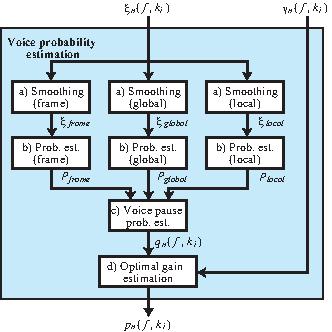Figure 6.78: Procedure for estimation of probability of speech presence:

Figure 6.78 shows the flow of estimation of probability of speech presence. Estimation of the probability of speech presence consists of:
a) Smoothing of the preliminary SNR for each of the 3 types of bands
b) Estimation with the temporal probability of speech presence based on the smoothed SNR in each band
c) Speech quiescent probability is estimated based on three provisional probability.
d) Estimation of the final probability of speech presence.

#### 6.3.11.5.8 3-a) Smoothing of preliminary SNR

The variables used in 3-a) are summarized in Table 6.72.

Table 6.72: Definition of variable
 Variable Description, corresponding parameter $\zeta _ n(k_ i)$ Time preliminary SNR temporally-smoothed $\xi _ n(k_ i)$ Preliminary SNR $\zeta ^{f}_ n(k_ i)$ Frequency-smoothed SNR (frame) $\zeta ^{g}_ n(k_ i)$ Frequency-smoothed SNR (global) $\zeta ^{l}_ n(k_ i)$ Frequency smoothing SNR (local) $b$ Parameter PRIOR_SNR_SMOOTH_FACTOR. The default value is 0.7 $F_{st}$ Parameter LOWER_SMOOTH_FREQ_INDEX. The default value is 8 $F_{en}$ Parameter UPPER_SMOOTH_FREQ_INDEX. The default value is 99 $G$ Parameter GLOBAL_SMOOTH_BANDWIDTH. The default value is 29 $L$ Parameter LOCAL_SMOOTH_BANDWIDTH. The default value is 5

First, temporally-smoothing is performed with the preliminary SNR $\xi _ n(f,k_ i)$ calculated by Equation (111) and the temporally-smoothed preliminary SNR $\zeta _ n(f-1,k_ i)$ of the former frame.

 $\displaystyle \zeta _ n(f,k_ i)$ $\displaystyle =$ $\displaystyle b \zeta _ n(f-1,k_ i)+ (1-b) \xi _ n(f,k_ i)$ (116)

Smoothing of the frequency direction is reduced in the order of frame, global, local depending on the size of the frame.

• Frequency smoothing in frame
Smoothing by averaging is performed in the frequency bin range $F_{st} \sim F_{en}$.

 $\displaystyle \zeta ^{f}_ n(k_ i)$ $\displaystyle =$ $\displaystyle \frac{1}{F_{en}-F_{st}+1}\sum _{k_ j=F_{st}}^{F_{en}}\zeta _ n(k_ j) \label{eq:SNR-smooth-frame}$ (117)
• Global frequency smoothing in global
Frequency smoothing with a hamming window in width $G$ is performed globally.

 $\displaystyle \zeta ^{g}_ n(k_ i)$ $\displaystyle =$ $\displaystyle \sum _{j=-(G-1)/2}^{(G-1)/2}w_{han}(j+(G-1)/2)\zeta _ n(k_{i+j}),$ (118) $\displaystyle w_{han}(j)$ $\displaystyle =$ $\displaystyle \frac{1}{C}\left(0.5 - 0.5 \cos \left( \frac{2 \pi j}{G}\right)\right),$ (119)

Here, $C$ is a normalization coefficient so that $\sum _{j=0}^{G-1} w_{han}(j) = 1$ can be satisfied.

• Local frequency smoothing
Frequency smoothing with a triangle window in width $F$ is performed locally.

 $\displaystyle \zeta ^{l}_ n(k_ i)$ $\displaystyle =$ $\displaystyle 0.25 \zeta _ n(k_ i-1)+ 0.5 \zeta _ n(k_ i)+ 0.25(k_ i+1)$ (120)

#### 6.3.11.5.9 3-b Estimation of the probability of provisional speech

The variables used in 3-b) are shown in Table 6.73.

Table 6.73: Definition of variable
 Variable Description, corresponding parameter $\zeta ^{f,g,l}_ n(k_ i)$ SNR smoothed in each band $P^{f,g,l}_ n(k_ i)$ Probability of provisional speech in each band $\zeta ^{peak}_ n(k_ i)$ Peak of smoothed SNR $Z^{peak}_{min}$ Parameter MIN_SMOOTH_PEAK_SNR. The default value is 1. $Z^{peak}_{max}$ Parameter MAX_SMOOTH_PEAK_SNR. The default value is 10. $Z_{thres}$ FRAME_SMOOTH_SNR_THRESH. The default value is 1.5. $Z_{min}^{f,g,l}$ Parameter MIN_FRAME_SMOOTH_SNR, MIN_GLOBAL_SMOOTH_SNR, MIN_LOCAL_SMOOTH_SNR. The default value is 0.1. $Z_{max}^{f,g,l}$ Parameter MAX_FRAME_SMOOTH_SNRF, MAX_GLOBAL_SMOOTH_SNR, MAX_LOCAL_SMOOTH_SNR. The default value is 0.316.
• Calculation of $P^{f}_ n(k_ i)$ and $\zeta ^{peak}_ n(k_ i)$
First, calculate $\zeta ^{peak}_ n(f,k_ i)$ as follows.

 $\displaystyle \zeta ^{peak}_ n(f,k_ i)$ $\displaystyle =$ $\displaystyle \left\{ \begin{array}{cr} \zeta ^ f_ n(f,k_ i),& \mathrm{if\ } \zeta ^{f}_ n(f,k_ i)> Z_{thres} \zeta ^ f_ n(f-1,k_ i)\\ \zeta ^{peak}_ n(f-1,k_ i),& \mathrm{if\ otherwise}. \end{array} \right.$ (121)

Here, the value of $\zeta ^{peak}_ n(k_ i)$ must be within the range of the parameter $Z^{peak}_{min},Z^{peak}_{max}$. That is,

 $\displaystyle \zeta ^{peak}_ n(k_ i)$ $\displaystyle =$ $\displaystyle \left\{ \begin{array}{cr} Z^{peak}_{min},& \mathrm{if} \ \zeta ^{peak}_ n(k_ i) Z^{peak}_{max} \end{array} \right.$ (122)

Next, $P_ n^ f(k_ i)$ is obtained as follows.

 $\displaystyle P^{f}_ n(k_ i)$ $\displaystyle =$ $\displaystyle \left\{ \begin{array}{cr} 0, & \mathrm{if}\ \zeta ^ f_ n(k_ i)< \zeta ^{peak}_ n(k_ i) Z_{min}^ f \\ 1, & \mathrm{if}\ \zeta ^ f_ n(k_ i)> \zeta ^{peak}_ n(k_ i) Z_{max}^ f\\ \frac{\log \left(\zeta ^ f_ n(k_ i)/ \zeta ^{peak}_ n(k_ i)Z^ f_{min}\right)}{\log \left( Z^ f_{max} / Z^ f_{min} \right)},& \mathrm{otherwise} \end{array} \right.$ (123)
• Calculation of $P^{g}_ n(k_ i)$
Calculate as follows.

 $\displaystyle P^ g_ n(k_ i)$ $\displaystyle =$ $\displaystyle \left\{ \begin{array}{cr} 0,& \mathrm{if}\ \ \zeta ^ g_ n(k_ i) < Z_{min}^ g\\ 1,& \mathrm{if}\ \ \zeta ^ g_ n(k_ i) > Z_{max}^ g\\ \frac{\ \log \left(\zeta ^ g_ n(k_ i)/ Z_{min}^ g\right)\ }{\ \log \left(Z_{max}^ g/ Z_{min}^ g\right)\ },& \mathrm{otherwise} \end{array} \right.$ (124)

Calculation of $P^{l}_ n(k_ i)$
Calculate as follows.

 $\displaystyle P^ l_ n(k_ i)$ $\displaystyle =$ $\displaystyle \left\{ \begin{array}{cr} 0,& \mathrm{if}\ \ \zeta ^ l_ n(k_ i) < Z_{min}^ l\\ 1,& \mathrm{if}\ \ \zeta ^ l_ n(k_ i) > Z_{max}^ l\\ \frac{\ \log \left(\zeta ^ l_ n(k_ i)/ Z_{min}^ l\right)\ }{\ \log \left(Z_{max}^ l/ Z_{min}^ l\right)\ },& \mathrm{otherwise} \end{array} \right.$ (125)

#### 6.3.11.5.10 3-c) Estimation of the probability of speech pause

The variables used in 3-c) are shown in Table 6.74.

Table 6.74: Definition of variable
 Variable description, a corresponding parameter $q_ n(k_ i)$ Probability of speech pause. $a^{f}$ FRAME_VOICEP_PROB_FACTOR. The default value is 0.7. $a^{g}$ GLOBAL_VOICEP_PROB_FACTOR. The default value is 0.9. $a^{l}$ LOCAL_VOICEP_PROB_FACTOR. The default value is 0.9. $q_{min}$ MIN_VOICE_PAUSE_PROB. The default value is 0.02. $q_{max}$ MAX_VOICE_PAUSE_PROB. The default value is 0.98.

As shown below, the probability of speech pause $q_ n(k_ i)$ is obtained by integrating the provisional probability of speech calculated from a smoothing result of the three frequency bands $P^{f,g,l}_ n(k_ i)$.

 $\displaystyle q_ n(k_ i)$ $\displaystyle =$ $\displaystyle 1 - \left( 1-a^ l+a^ l P^ l_ n(k_ i) \right) \left( 1-a^ g +a^ g P^ g_ n(k_ i) \right) \left( 1-a^ f+ a^ f P^ f_ n(k_ i) \right),$ (126)

Here, when $q_ n(k_ i) < q_{min}$, $q_ n(k_ i) = q_{min}$, and when $q_ n(k_ i) > q_{max}$, $q_ n(k_ i) = q_{max}$.

#### 6.3.11.5.11 3-d) Estimation of the probability of speech presence

The probability of speech presence $p_ n(k_ i)$ is obtained by the probability of speech suspension pause $q_ n(k_ i)$, the preliminary SNR $\zeta _ n(k_ i)$ and the intermediate variable $v_ n(k_ i)$ derived by Equation (113).

 $\displaystyle p_ n(k_ i)$ $\displaystyle =$ $\displaystyle \left\{ 1 + \frac{q_ n(k_ i)}{1-q_ n(k_ i)} \left( 1+\zeta _ n(k_ i)\right) \exp \left(-v_ n(k_ i)\right)\right)^{-1}$ (127)

4 Noise removal:

The enhanced separated sound as an output ${\hat S}_ n(k_ i)$ is derived by activating the optimal gain $G^{H1}_ n(k_ i)$ and the probability of speech presence $p_ n(k_ i)$ for the separated sound spectrum as the input $Y_ n(k_ i)$.

 $\displaystyle {\hat S}_ n(k_ i)$ $\displaystyle =$ $\displaystyle Y_ n(k_ i) G^{H1}_ n(k_ i) p_ n(k_ i)$ (128)